• t=0:23; a=random('poisson',700,1,24); %原图 plot(t,a,'b') hold on; %1.拟合 c=polyfit(t,a,2); d=polyval(c,t,1); plot(t,d,'r') %2.spcrv values = spcrv([[t(1) t t(end)];[a(1) a a(end)]],3);...
t=0:23;
a=random('poisson',700,1,24);
%原图
plot(t,a,'b')
hold on;
%1.拟合
c=polyfit(t,a,2);
d=polyval(c,t,1);
plot(t,d,'r')
%2.spcrv
values = spcrv([[t(1) t t(end)];[a(1) a a(end)]],3);
plot(values(1,:),values(2,:), 'g');



展开全文matlab
• 本期小编将为大家浅谈一下优美的...贝塞尔曲线可以用来创建平滑曲线的道路、弯曲的路径就像 祖玛游戏、弯曲型的河流等。我们在做游戏开发的时候，例如像球类游戏，球的运动轨迹，就可以用贝塞尔曲线来完美展现 ...
本期小编将为大家浅谈一下优美的贝塞尔曲线(ˉ▽￣～)   ~

以下内容来自网上学习总结

一、简介
1.简单介绍一下什么是贝塞尔曲线 (*￣︶￣)  以下几点总结：

贝塞尔曲线是最基本的曲线，一般用在计算机 图形学和 图像处理。贝塞尔曲线可以用来创建平滑的曲线的道路、弯曲的路径就像 祖玛游戏、弯曲型的河流等。我们在做游戏开发的时候，例如像球类游戏，球的运动轨迹，就可以用贝塞尔曲线来完美展现 (ˉ▽￣～)

一条贝塞尔曲线是由一组定义的控制点 P0到 Pn，在 n 调用它的顺序 (n = 1 为线性，2 为二次，等.)。第一个和最后一个控制点总是具有终结点的曲线;然而，中间两个控制点 (如果有的话) 一般不会位于曲线上 。 （是不是有点蒙）

（1）贝塞尔曲线包含两个控制点即 n = 2 称为线性的贝塞尔曲线

（2）贝塞尔曲线包含三个控制点即 n = 3 称为二次贝塞尔曲线

（3）贝塞尔曲线包含四个控制点即 n = 4，所以称为三次贝塞尔曲线。

贝塞尔曲线返回点的贝塞尔函数，使用线性插值的概念作为基础。

后期更新~~~


展开全文• 上一篇文章我们通过getLineChartView来实现了折线...而通过查看API我们发现achartengine还提供了一个getCubeLineChartView来获取一个显示平滑曲线的view，使用起来和获取普通折线图没有太大的区别ChartFactory.getCubeL
上一篇文章我们通过getLineChartView来实现了折线图的显示

ChartFactory.getLineChartView(mContext, dataset, renderer);

而通过查看API我们发现achartengine还提供了一个getCubeLineChartView来获取一个显示平滑曲线的view，使用起来和获取普通折线图没有太大的区别

ChartFactory.getCubeLineChartView(mContext, dataset, renderer, 0.3F);

这里多了最后一个设置平滑度的系数，效果图如下：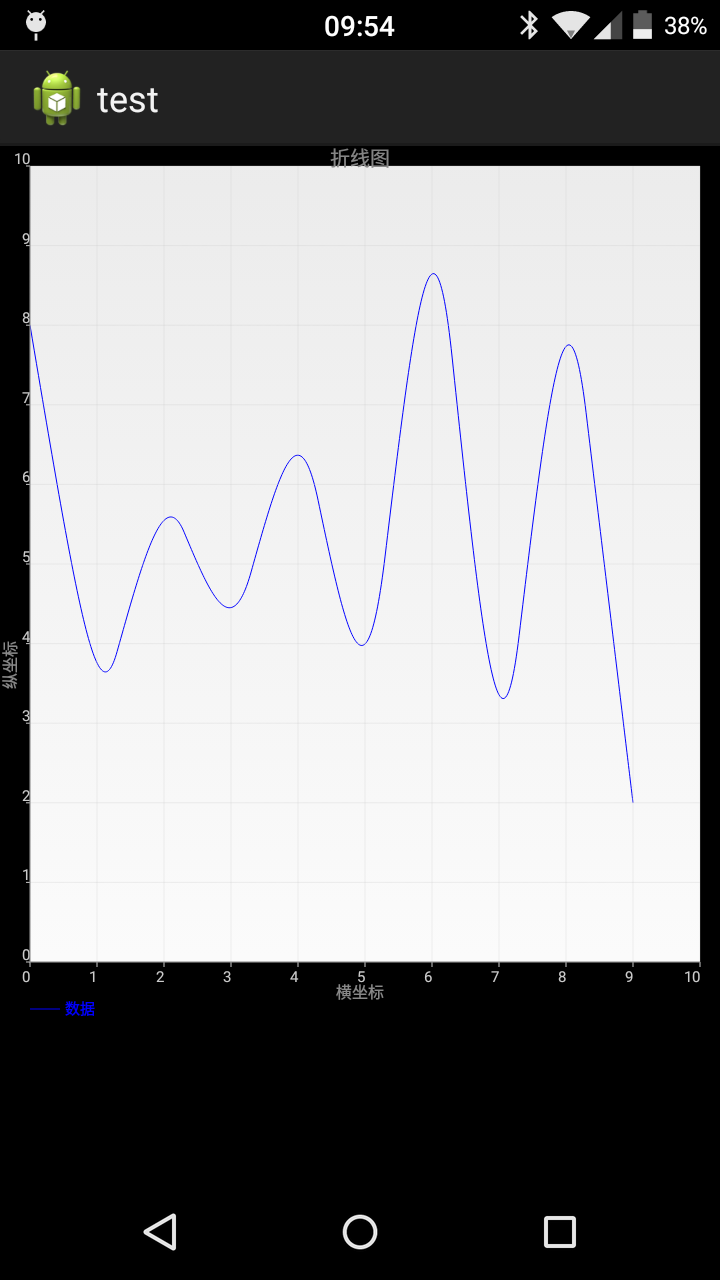通过观察效果图我们发现曲线并没有经过我们设置的点，怎么回事，我们的程序有BUG了？显然并不是。在解释这个情况之前，我们需要理解一个概念：贝塞尔曲线

这里我假设你已经大概浏览了维基百科的内容，因此我们知道贝塞尔曲线必过两个端点，并且受控制点控制，achartengine在绘制平滑曲线的时候使用的三阶贝塞尔曲线（安卓在Path.java这个类中只提供了二阶quadTo和三阶cubicTo贝塞尔曲线），这里引用一下维基百科上的图片可以更加直观的展现三阶贝塞尔曲线的绘制过程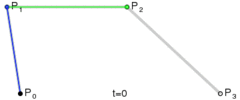通过观察上图我们发现三阶贝塞尔曲线必过P0和P3点，受P1和P2点控制，好了概念介绍完毕，我们就可以来解释一下为什么achartengine的平滑曲线没有经过数据点了。

在achartengine的源码CubicLineChart.java中

  protected void drawPath(Canvas canvas, List<Float> points, Paint paint, boolean circular) {
Path p = new Path();
float x = points.get(0);
float y = points.get(1);
p.moveTo(x, y);

int length = points.size();
if (circular) {
length -= 4;
}

Point p1 = new Point();
Point p2 = new Point();
Point p3 = new Point();
for (int i = 0; i < length; i += 2) {
int nextIndex = i + 2 < length ? i + 2 : i;
int nextNextIndex = i + 4 < length ? i + 4 : nextIndex;
calc(points, p1, i, nextIndex, mSecondMultiplier);
p2.setX(points.get(nextIndex));
p2.setY(points.get(nextIndex + 1));
calc(points, p3, nextIndex, nextNextIndex, mFirstMultiplier);
// From last point, approaching x1/y1 and x2/y2 and ends up at x3/y3
p.cubicTo(p1.getX(), p1.getY(), p2.getX(), p2.getY(), p3.getX(), p3.getY());
}
...//省略部分代码
canvas.drawPath(p, paint);
}

其中P对应上图中的P0，而P1,P2,P3依次对应贝塞尔曲线的P1,P2,P3，这里需要说明一下的是，P1和P3是根据平滑度系数计算得出的点，而P2则是我们自己设置的点，根据贝塞尔曲线公式，曲线是不会经过P2的，因为我们的数据点在贝塞尔曲线中充当了控制点的角色。这就解释了为什么我们使用getCubeLineChartView的时候曲线没有经过数据点。
有些聪明的同学可能会立即反应，如果我们将数据点设置为曲线的端点就可以让曲线经过数据点了，因为贝塞尔曲线是必过端点的，这里我不建议这样做，因为这样绘制出来的曲线会非常难看，喜欢动手的同学可以自己试一下
展开全文• 很多文章在谈及曲线平滑的时候，习惯使用拟合的概念，我认为这是不恰当的。平滑后的曲线，一定经过原始的数据点，而拟合曲线，则不一定要经过原始数据点。 一般而言，需要平滑的数据分为两种：时间序列的单值数据、...
文章目录前言贝塞尔曲线算法描述算法实现参考资料
前言
很多文章在谈及曲线平滑的时候，习惯使用拟合的概念，我认为这是不恰当的。平滑后的曲线，一定经过原始的数据点，而拟合曲线，则不一定要经过原始数据点。
一般而言，需要平滑的数据分为两种：时间序列的单值数据、时间序列的二维数据。对于前者，并非一定要用贝塞尔算法，仅用样条插值就可以轻松实现平滑；而对于后者，不管是 numpy 还是 scipy 提供的那些插值算法，就都不适用了。
本文基于三阶贝塞尔曲线，实现了时间序列的单值数据和时间序列的二维数据的平滑算法，可满足大多数的平滑需求。
贝塞尔曲线
关于贝塞尔曲线的数学原理，这里就不讨论了，直接贴出结论：

一阶贝塞尔曲线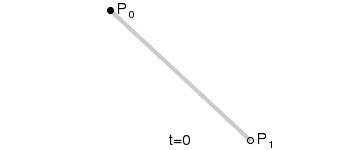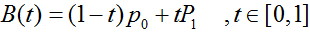二阶贝塞尔曲线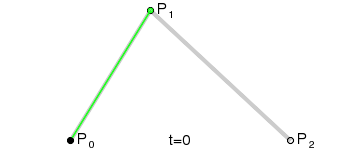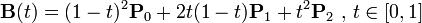三阶贝塞尔曲线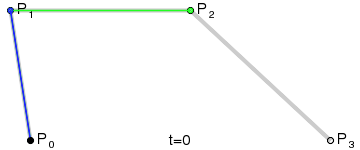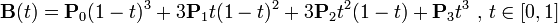算法描述
如果我们把三阶贝塞尔曲线的 P0 和 P3 视为原始数据，只要找到 P1 和 P2 两个点（我们称其为控制点），就可以根据三阶贝塞尔曲线公式，计算出 P0 和 P3 之间平滑曲线上的任意点。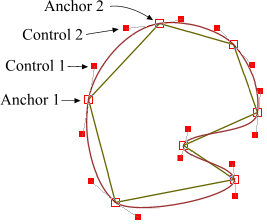现在，平滑问题变成了如何计算两个原始数据点之间的控制点的问题。步骤如下：
第1步：绿色直线连接相邻的原始数据点，计算出个线段的中点，红色直线连接相邻的中点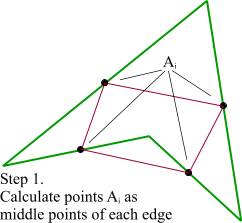第2步：根据相邻两条绿色直线长度之比，分割其中点之间红色连线，标记分割点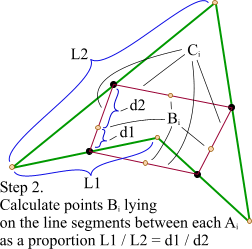第3步：平移红色连线，使其分割点与相对的原始数据点重合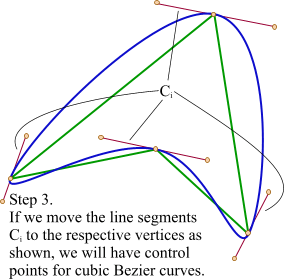第4步：调整平移后红色连线的端点与原始数据点的距离，通常缩减40%-80%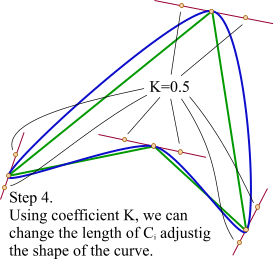算法实现
# -*- coding: utf-8 -*-

import numpy as np

def bezier_curve(p0, p1, p2, p3, inserted):
"""
三阶贝塞尔曲线

p0, p1, p2, p3  - 点坐标，tuple、list或numpy.ndarray类型
inserted        - p0和p3之间插值的数量
"""

assert isinstance(p0, (tuple, list, np.ndarray)), u'点坐标不是期望的元组、列表或numpy数组类型'
assert isinstance(p0, (tuple, list, np.ndarray)), u'点坐标不是期望的元组、列表或numpy数组类型'
assert isinstance(p0, (tuple, list, np.ndarray)), u'点坐标不是期望的元组、列表或numpy数组类型'
assert isinstance(p0, (tuple, list, np.ndarray)), u'点坐标不是期望的元组、列表或numpy数组类型'

if isinstance(p0, (tuple, list)):
p0 = np.array(p0)
if isinstance(p1, (tuple, list)):
p1 = np.array(p1)
if isinstance(p2, (tuple, list)):
p2 = np.array(p2)
if isinstance(p3, (tuple, list)):
p3 = np.array(p3)

points = list()
for t in np.linspace(0, 1, inserted+2):
points.append(p0*np.power((1-t),3) + 3*p1*t*np.power((1-t),2) + 3*p2*(1-t)*np.power(t,2) + p3*np.power(t,3))

return np.vstack(points)

def smoothing_base_bezier(date_x, date_y, k=0.5, inserted=10, closed=False):
"""
基于三阶贝塞尔曲线的数据平滑算法

date_x      - x维度数据集，list或numpy.ndarray类型
date_y      - y维度数据集，list或numpy.ndarray类型
k           - 调整平滑曲线形状的因子，取值一般在0.2~0.6之间。默认值为0.5
inserted    - 两个原始数据点之间插值的数量。默认值为10
closed      - 曲线是否封闭，如是，则首尾相连。默认曲线不封闭
"""

assert isinstance(date_x, (list, np.ndarray)), u'x数据集不是期望的列表或numpy数组类型'
assert isinstance(date_y, (list, np.ndarray)), u'y数据集不是期望的列表或numpy数组类型'

if isinstance(date_x, list) and isinstance(date_y, list):
assert len(date_x)==len(date_y), u'x数据集和y数据集长度不匹配'
date_x = np.array(date_x)
date_y = np.array(date_y)
elif isinstance(date_x, np.ndarray) and isinstance(date_y, np.ndarray):
assert date_x.shape==date_y.shape, u'x数据集和y数据集长度不匹配'
else:
raise Exception(u'x数据集或y数据集类型错误')

# 第1步：生成原始数据折线中点集
mid_points = list()
for i in range(1, date_x.shape):
mid_points.append({
'start':    (date_x[i-1], date_y[i-1]),
'end':      (date_x[i], date_y[i]),
'mid':      ((date_x[i]+date_x[i-1])/2.0, (date_y[i]+date_y[i-1])/2.0)
})

if closed:
mid_points.append({
'start':    (date_x[-1], date_y[-1]),
'end':      (date_x, date_y),
'mid':      ((date_x+date_x[-1])/2.0, (date_y+date_y[-1])/2.0)
})

# 第2步：找出中点连线及其分割点
split_points = list()
for i in range(len(mid_points)):
if i < (len(mid_points)-1):
j = i+1
elif closed:
j = 0
else:
continue

x00, y00 = mid_points[i]['start']
x01, y01 = mid_points[i]['end']
x10, y10 = mid_points[j]['start']
x11, y11 = mid_points[j]['end']
d0 = np.sqrt(np.power((x00-x01), 2) + np.power((y00-y01), 2))
d1 = np.sqrt(np.power((x10-x11), 2) + np.power((y10-y11), 2))
k_split = 1.0*d0/(d0+d1)

mx0, my0 = mid_points[i]['mid']
mx1, my1 = mid_points[j]['mid']

split_points.append({
'start':    (mx0, my0),
'end':      (mx1, my1),
'split':    (mx0+(mx1-mx0)*k_split, my0+(my1-my0)*k_split)
})

# 第3步：平移中点连线，调整端点，生成控制点
crt_points = list()
for i in range(len(split_points)):
vx, vy = mid_points[i]['end'] # 当前顶点的坐标
dx = vx - split_points[i]['split'] # 平移线段x偏移量
dy = vy - split_points[i]['split'] # 平移线段y偏移量

sx, sy = split_points[i]['start']+dx, split_points[i]['start']+dy # 平移后线段起点坐标
ex, ey = split_points[i]['end']+dx, split_points[i]['end']+dy # 平移后线段终点坐标

cp0 = sx+(vx-sx)*k, sy+(vy-sy)*k # 控制点坐标
cp1 = ex+(vx-ex)*k, ey+(vy-ey)*k # 控制点坐标

if crt_points:
crt_points[-1].insert(2, cp0)
else:
crt_points.append([mid_points['start'], cp0, mid_points['end']])

if closed:
if i < (len(mid_points)-1):
crt_points.append([mid_points[i+1]['start'], cp1, mid_points[i+1]['end']])
else:
crt_points.insert(1, cp1)
else:
if i < (len(mid_points)-2):
crt_points.append([mid_points[i+1]['start'], cp1, mid_points[i+1]['end']])
else:
crt_points.append([mid_points[i+1]['start'], cp1, mid_points[i+1]['end'], mid_points[i+1]['end']])
crt_points.insert(1, mid_points['start'])

# 第4步：应用贝塞尔曲线方程插值
out = list()
for item in crt_points:
group = bezier_curve(item, item, item, item, inserted)
out.append(group[:-1])

out.append(group[-1:])
out = np.vstack(out)

return out.T, out.T

if __name__ == '__main__':
import matplotlib.pyplot as plt

x = np.array([2,4,4,3,2])
y = np.array([2,2,4,3,4])

plt.plot(x, y, 'ro')
x_curve, y_curve = smoothing_base_bezier(x, y, k=0.3, closed=True)
plt.plot(x_curve, y_curve, label='$k=0.3$')
x_curve, y_curve = smoothing_base_bezier(x, y, k=0.4, closed=True)
plt.plot(x_curve, y_curve, label='$k=0.4$')
x_curve, y_curve = smoothing_base_bezier(x, y, k=0.5, closed=True)
plt.plot(x_curve, y_curve, label='$k=0.5$')
x_curve, y_curve = smoothing_base_bezier(x, y, k=0.6, closed=True)
plt.plot(x_curve, y_curve, label='$k=0.6$')
plt.legend(loc='best')

plt.show()

下图为平滑效果。左侧是封闭曲线，两个原始数据点之间插值数量为默认值10；右侧为同样数据不封闭的效果，k值默认0.5.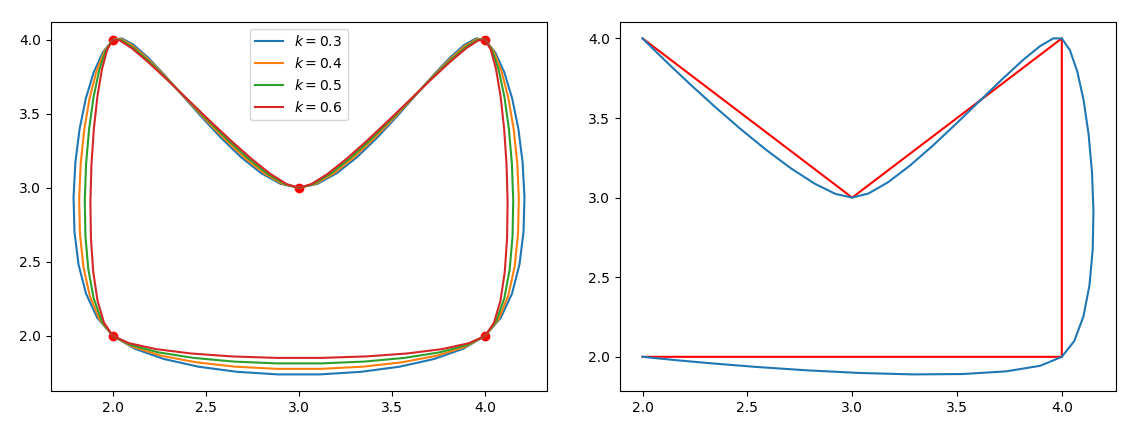参考资料
算法参考了 Interpolation with Bezier Curves 这个网页，里面没有关于作者的任何信息，在此只能笼统地向国际友人表示感谢！


展开全文贝塞尔
• python 数据、曲线平滑处理——方法总结Savitzky-Golay 滤波器实现曲线平滑插值法对折线进行平滑曲线处理基于Numpy.convolve实现滑动平均滤波数据平滑处理——log()和exp()函数 问题描述： 在寻找曲线的波峰、波谷时...数据分析
• 在二元分类中，精确召回曲线 (PRC) 已成为评估分类性能的广泛概念工具。 该曲线将分类器的阳性预测值与其真阳性率相关联，并为众所周知的接收者操作特征 (ROC) 提供了一个有用的替代方案。 可以根据有关基本决策值的...matlab
• 文章目录一、净额成交量二、标量函数矢量化三、数据曲线平滑 一、净额成交量 1、案例分析 符号数组a: [10 -20 30 40 -50] 用法：numpy.sign(a) 结果是[1 -1 1 1 -1] 净额成交量：简称OBV，赚了是正，赔了是负 利用...python numpy 数据可视化 数据分析 数学建模
• 16S结题报告中都会有这么一张图： 这张图是OTU Rank曲线，该曲线可以展示样品的多样性。而样品的多样性常通过以下两个方面进行解释：物种的丰富程度和均匀程度。Rank曲线中，曲线在横轴上的跨度越长...若曲线平滑...
• ## 贝塞尔曲线

千次阅读 2016-05-10 19:18:14
为什么可以做到如上两点，看下面的讲解：首先来说，贝塞尔曲线有阶的概念，这个阶可以理解为控制点，一阶的控制点只有两个。如上是一阶的方程，其中t取值为0到1，可以理解为时间，从开始到结束。动图如下：图中可以...贝塞尔 android 绘制 bezier
• ROC（Receiver Operating Characteristic，接受者工作特征曲线曲线和AUC常被用来评价一个二值分类器（binary classifier）的优劣。博文介绍ROC和AUC的特点，讨论如何作出ROC曲线图以及计算AUC。 AUC是现...roc
• 样条曲线被广泛应用于模型的几何重构平滑中。 文章目录样条曲线（Spline Curves）0. 基本概念1. 起源2. 贝塞尔曲线（Bézier curve）1.1 直线段表示（2 points）1.2 抛物线三切线定理（3 points）1.3 通用公式1.4 ...python 算法 机器学习 人工智能
• 游戏开发中的贝塞尔曲线，...它们依赖于插值（我在上一篇文章中提过），结合了多个步骤以创建平滑曲线。为了更好地了解贝塞尔曲线的工作原理，让我们从其最简单的形式开始：二次贝塞尔曲线。 二次贝塞尔曲线 取三点，游戏开发
• ## Gamma曲线

千次阅读 2015-01-12 15:41:03
问题：什么是Gamma曲线矫正？Gamma曲线矫正是什么意思？ Gamma 曲线是一种特殊的色调曲线，当Gamma值等于1的时候，曲线为与坐标轴成45°的直线，这个时候表示输入和输出密度相同。高于1的Gamma值将会造成 输出亮化...c++ cpu 显卡
• 目录1 概念1.1 核平滑概念和计算1.2 Nadaraya-Watson回归1.3 高斯核2 高斯核平滑过程-Python实现2.1 加载库和生成数据2.2 Full Width at Half Maximum (FWHM)2.3 分步进行平滑2.4 二维平滑2.5 为什么要进行平滑 ...机器学习
• 样条曲线、贝赛尔曲线 本文是Three.js电子书的7.3节 规则的曲线比如圆、椭圆、抛物线都可以用一个函数去描述，对于不...样条曲线、贝赛尔曲线样条曲线贝赛尔曲线经过一系列点创建平滑的样条曲线SplineCurve2DCatm...
• 很久之前就久闻该线大名，但是一直不是很了解，在经过一番谷歌之后，有了初步的概念：三点确定一条曲线：起点，终点，辅助点。 三个点的基本关系如下： 当初看这图我也看了老半天，只知道是非常平滑，不知道三个...Android 自定义view 进阶
• ## 滤波 平滑 去噪

千次阅读 2019-01-06 22:23:03
做动词 - 使平滑化：使信号变得具有平滑的属性 3. 去噪：一种具体应用，用有噪音污染的信号猜测干净的信号。 他们的联系？ 一句话给串起来： 要降噪，常用滤波来实现，平且常用低通滤波，副作用是往往使得信号...
• 前言 在最近的一个项目中做了一个涂鸦的效果，手指快速移动，会出现折线，这篇文章记录笔触优化。...这里用的到有个叫贝塞尔曲线概念。关于贝塞尔曲线公式，它是依据几个位置任意的点坐标绘制出的一条光滑曲线
• ## Unity游戏中使用贝塞尔曲线

万次阅读 多人点赞 2015-08-18 09:08:14
孙广东 2015.8.15比如在3D rpg游戏中，我们想设置弹道，不同的轨迹类型...贝塞尔曲线可以用来创建平滑曲线的道路、 弯曲的路径就像 祖玛游戏、 弯曲型的河流等。 一条贝塞尔曲线是由一组定义的控制点 P0到 Pn，在 ncamera unity unity3d
• Bezier曲线理论与OpenGL实现 在专栏的第二节中，我简单梳理了计算机图形学当中简单的数理知识和常见的图形学概念。...在绘图术语中，**样条是通过一组指定点集而生成平滑曲线的柔性带。**当绘制曲线时，...opengl
• ## 浅谈贝塞尔曲线

千次阅读 2019-08-05 22:20:02
浅谈贝塞尔曲线 前段时间做了一个手写板功能的...概念 在数学的数值分析领域中，贝塞尔曲线（英语：Bézier curve，亦作“贝塞尔”）是计算机图形学中相当重要的参数曲线。更高维度的广泛化贝塞尔曲线就称作贝兹曲面...Android
• 1贝塞尔曲线简介 ...应用：抛物线，水波纹，平滑曲线。 具体数学推导公式我也看不懂，只能给大家贴两个网上大神制作的贝塞尔曲线生成过程动画。这里列出的公式后面会用到。 二维贝塞尔曲线公式：...quadTo
• Unity 工具类 之 贝塞尔 Bezier 曲线 目录 Unity 工具类 之 贝塞尔 Bezier 曲线 一、简单介绍 二、原理与分类 ...六、样例使用步骤（三次...贝塞尔曲线可以用来创建平滑曲线的道路、 弯曲的路径就像 祖玛游戏...Unity 工具类 贝塞尔
• 现代扫描技术的发展使得获取点云数据不再困难，通过曲线重建技术可以获取表面网格来表示各种复杂的实体。但是点云数据中往往存在噪声，这样得到的重建网格通常都需要进行平滑处理。 拉普拉斯平滑是一种常用的网格...
• 滤波是信号处理中的概念，指的是将信号中特定波段频率滤除的操作。在图像处理中，和通过傅里叶变化实现的频域下的滤波是等效的，所以也称为滤波。首先要学习的是空间域滤波，主要是基于邻域(空间域)对图像中的像素...
• ## ROC曲线与PR曲线

千次阅读 2017-07-22 22:27:20
之前一直知道怎么看ROC，没有过深理解相关概念（真阳假阳等）最近重新细看ROC和PR发现很多资料把这些概念搞得乱七八糟的，所以围绕ROC和PR阐述一下，并且比较两者在评价模型时的优劣。 一、概念介绍 1、混淆矩阵 2...
• 贝塞尔曲线可以用来创建平滑曲线的道路、 弯曲的路径就像 祖玛游戏、 弯曲型的河流等。 贝塞尔曲线：（贝塞尔曲线的基本想法部分摘自http://blog.csdn.net/u010019717/article/details/47684223 。仅供学习，知识...unity3d...